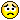# Why the complicated Solve output?

Posted 9 years ago
1412 Views
|
4 Replies
|
0 Total Likes
|
 Hi to all's,the problem:The second is ok while the first ...Why?RegardsStefan
4 Replies
Sort By:
Posted 9 years ago
 Thanks again!Mathematica 9.0.1 on XP 32 bitNowk = 2In:= Reduce[(x + k)^2/3 + (3 x + k)/2 < (2 x - 7)/2 - (x - 5)/2 + 3 k , x]Out= > -(1/8) && -(7/2) - (3 Sqrt[1 + 8])/2 < x < -(7/2) + (3 Sqrt[1 + 8])/2orIn:= Reduce[(x + k)^2/3 + (3 x + k)/2 - (2 x - 7)/2 + (x - 5)/2 - 3 k < 0 , x]Out= -8 < x < 1Stefan
Posted 9 years ago
 Mathematica 9.0.1 on Windows 7 64 bit does itIn:= Reduce[(2 x + 1)^2 - (x - 3)^2 >= 2 (x - 1) (x + 2) - 11, x]Out= x <= -7 || x >= -1ordering an inequality forces x to be an integer, rational number or a real one. What happens if you state the tautology in your versionIn:= Reduce[(2 x + 1)^2 - (x - 3)^2 >= 2 (x - 1) (x + 2) - 11, x, Reals]Out= x <= -7 || x >= -1
Posted 9 years ago
 Thanks,I must solve too an inequality and there is same problem!StefanAttachments:
Posted 9 years ago
 The first is exactly the same as the second by the way, if you are concerned with the representation, use SimplifyIn:= Solve[(2 x + 1)^2 - (x - 3)^2 == 2 (x - 1) (x + 2) - 11, x] // SimplifyOut= {{x -> -7}, {x -> -1}}